## research papersJOURNAL OFSYNCHROTRONRADIATION
ISSN: 1600-5775
Volume 26| Part 4| July 2019| Pages 1260-1265

## Temperature-induced molecule assembly effects on the near-edge X-ray absorption fine-structure spectra of Zn-phthalocyanine layers on Si substrates

aHelmholtz-Zentrum Berlin für Materie und Energie, Hahn-Meitner-Platz 1, 14109 Berlin, Germany, and bCentre for Storage Ring Facilities (ISA), Department of Physics and Astronomy, Ny Munkegade 120, Building 1525, 326 Aarhus, Denmark
*Correspondence e-mail: fostiropoulos@helmholtz-berlin.de

(Received 24 August 2018; accepted 2 April 2019; online 21 May 2019)

The molecular arrangement of vacuum thermally deposited polycrystalline Zn-phthalocyanine (ZnPc) layers on Si substrates is investigated using near-edge X-ray absorption fine-structure (NEXAFS) spectroscopy in the proximity of the carbon edge at E0 = 287.33 eV. The data were collected as a function of the deposition substrate temperature TS (30, 90, 150°C) and the incidence angle θ (20°, 45°, 70°, 90°) of the synchrotron beam with respect to the sample plane. Each spectrum was analysed by mathematical simulation applying an error function for the carbon edge and a set of Voigt and (asymmetric) Gaussian functions for C1sπ* and C1sσ* transitions of ZnPc, respectively. It turned out that part of the organic layer consists of adventitious carbon, which does not contribute to the molecular transitions of ZnPc, whereas all molecular features exhibit polarization-dependent peak areas pointing to a reasonable fraction of well-assembled molecules at any TS. The highest adventitious carbon fraction was found at TS = 30°C, whereas the highest polarization dependence was found at TS = 90°C. The calculated average molecular tilt angles for the three temperatures (30, 90, 150°C) were γ = 60.6°, 68.7° and 66.7°, respectively. If only the polarization-dependent fractions are considered, then the three samples can be mathematically described using a shared molecular tilt angle of γ = 68.7°, which corresponds to the average tilt angle of the TS = 90°C sample.

### 1. Introduction

The transport properties of molecular polycrystalline materials are determined by the degree of molecular order and orientation (Lunt et al., 2009; Münch, 2001; de Oteyza et al., 2005; Ostrick et al., 1997). For planar aromatic molecules like metal phthalocyanines, charge transport in the crystallites is highly anisotropic (Ostrick et al., 1997; Babajanyan et al., 2011; Hamann, 1978; Michaelis et al., 2004; Mathur & Ramesh, 1976) and conductivity is favoured in the direction of intermolecular ππ overlap, i.e. perpendicular to the molecules' planes (Chen et al., 2001; Bao, 2000). Because in many applications conductivity in a given direction is required, it is important to know what influences the molecular orientation and the crystallites' growth direction.

Metal phthalocyanine molecules have been studied in the past because of their role as absorber in organic solar cells (Fostiropoulos et al., 2003). They form a variety of crystalline and non-crystalline structures depending on the preparation conditions. Commercially available sublimed powders consist of well-crystallized needles of a few millimetres in length with aspect ratios in the order up to 1:100 and even more.

In vacuum deposition processes we have grown tiny, spherical, mainly amorphous clusters of a few nanometres in size on room-temperature substrates (Fig. 1, left). Flat lying elongated crystallites were obtained on annealed substrates (Fig. 1, centre), whereas, if an excessive amount of material is deposited, then the crystallites grow as spaghetti-like fibres out of the plane (Fig. 1, right).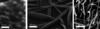Figure 1 Scanning electron micrographs of vacuum thermal deposited phthalocyanine grown on metal oxides. Left: semi-spherical molecular clusters grown on indium tin oxide at room temperature. Centre: tagliatelle'- as well as french fries'-shaped molecular crystallites formed on SiOx at 210°C. Right: spaghetti-like fibres grow on annealed SiOx when excessive material is deposited.

The molecules in flat lying structures like in Fig. 1, centre, are reported to `stand' roughly upright on metal oxide substrates, influenced by the organic–inorganic interface (Peisert et al., 2009, 2001; Biswas et al., 2009). The influence of the layer thickness has been investigated but effects of the substrate temperature on the molecular orientation have not yet been addressed. However, such temperature effects have an impact on optical (Greiner et al., 2013) and electrical layer properties and the performance of related organic electronic devices such as small-molecule organic solar cells (Vogel et al., 2006; Fostiropoulos & Schindler, 2009).

In this paper we study temperature-induced arrangements of Zn-phthalocyanine (ZnPc) molecules in self-assembled layers on native oxide coated Si wafers by means of polarization-dependent near-edge X-ray absorption fine-structure (NEXAFS) spectroscopy. Data were collected at the carbon K-edge from 280 to 320 eV using the surface-sensitive partial Auger electron yield modus. Effects of the substrate temperature during deposition on the molecular arrangement are demonstrated.

### 2. Experiments and data simulation

Since phthalocyanine molecules tend to form islands of molecular stacks (Figs. 1and 2) instead of flat monolayers, we deposited approximately 3 nm (approximately two monolayers) to achieve enough surface coverage for the NEXAFS analysis. The layers were prepared by thermal evaporation of ZnPc powder under a pressure of <10−7 mbar vacuum at a deposition rate of ∼1.0 Å s−1 onto a 1 nm SiOx layer on Si at substrate temperatures TS = 30, 90 and 150°C. The film thickness was monitored using a quartz deposition controller, which was calibrated by X-ray reflectivity measurements. Prior to the deposition, the Si substrates had been washed in a supersonic bath with acetone, ethanol and distilled water. The surface roughness of the substrates was 0.3 nm, which was determined by atomic force microscopy. NEXAFS measurements were performed using linearly polarized X-rays at the ASTRID SX-700 beamline of ISA in Aarhus, Denmark, with a monochromator resolution ΔE = 0.88 eV.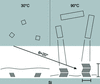Figure 2 Arrangement of ZnPc molecules deposited at T = 30°C and T = 90°C under vacuum conditions. At T = 30°C ZnPc forms mainly amorphous films with small crystalline domains. At T = 90°C these domains grow to long crystalline fibres lying on the substrate. All molecules contribute to the NEXAFS features that determine the average molecular tilt angle with respect to the substrate's plane.

For each sample, spectra at four synchrotron beam incident angles θ = 20°, 45°, 70° and 90° with respect to the substrates' plane were recorded. The spectra were mathematically described by the following simulation fit function: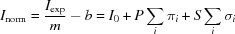with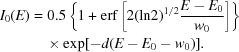We took into account the fact that for the experimental data Iexp the monochromator resolution ΔE dominates the shapes of the spectral features. Therefore, the step function I0 has been described in accordance with the work of Stoehr (1992) by a cumulative distribution function (CDF) with exponential decay (decay coefficient d = 6.08 for E > E0 + w0 else d = 0).

The molecular bands were fitted by peak sets πi and σi. Each πi set consists of a number of linear-dependent Voigt functions and each σi set consists of a number of linear-dependent asymmetric Gaussians with a full width at half-maximum (FWHM) given by a linear function of the photon energy (Stoehr, 1992). The best fit was achieved with the widths given in Table 1.

 Table 1Widths of molecular features and carbon edge
Features Gaussian width Lorentzian width
πi (Voigt) 0.74 ± 0.02 eV 0.21 ± 0.04 eV
I0 (erf) 3.41 ± 1.2 eV
σi (asymmetric Gaussian) 0.21 (E − 285.32) eV

For all calculated spectra, peak positions and widths, relative peak areas as well as the carbon edge position and width were shared fitting parameters. On the other hand, independent fitting parameters were the total areas P and S of the peak sets as well as the normalization parameters m and b. In this article only normalized data (Inorm) are presented.

### 3. Results and discussion

#### 3.1. Carbon edge

Commonly, C 1s NEXAFS spectra of aromatic systems like ZnPc are described (with increasing photon energy) in terms of a pre-edge, transitions into π* states below the edge, the absorption edge starting at the ionization energy of the C 1s state, and transitions into σ* states above the edge (Stoehr, 1992).

As expected, the collected 11 NEXAFS spectra could be simulated using the same position E0 and width w0 for their respective CDF functions. Fig. 3shows the normalized spectra, where the graphs were baseline corrected by subtracting a linear function and scaled to equalize the calculated edge intensities. Apparently, the normalized CDF do not depend on experimental parameters, i.e. preparation temperature TS and incidence angle θ. The calculated best-fit parameters for the applied error function are E0 = 287.33 ± 0.05 and w0 = 3.41 ± 0.12 eV, which is four times the detector resolution ΔE, indicating that there is more than one edge and, hence, chemically different carbon species.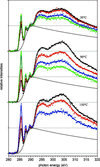Figure 3 Polarization-dependent NEXAFS spectra of ZnPc layers on Si deposited at substrate temperatures TS = 30, 90, 150°C and measured under incidence angles θ = 20°, 45°, 70°, 90°. All spectra were mathematically described by the same set of independent and shared parameters (coloured solid lines). For comparison the individual graphs have been scaled to equalize I0 of all carbon edges (black CDF curves).

#### 3.2. Molecular features

After subtraction of the simulated edge, the polarization-dependent features of the spectra can be displayed (Fig. 4). In the range below E0 a set of five molecular absorption bands (πi) at 284.85, 285.64, 286.23, 287.66 and 289.6 eV were detected (Table 2). They originate from resonant C1sπ* transitions of metal phthalocyanines (Zhang et al., 2005; Ikame et al., 2005; Okudaira et al., 2004). Their calculated (Voigt) peak widths are equal in all spectra. They consist of a Gauss and a Lorentz fraction of 0.74 and 0.21, respectively, which is in the range of the monochromator resolution.

 Table 2Positions and relative amplitudes of ZnPc π* transitions (A–E) are shared fit parameters
π* transitions A B C D E
Position (eV) 284.85 285.64 286.23 287.66 289.60
Relative amplitude 0.45 1 0.14 0.29 0.10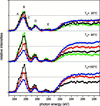Figure 4 π* transitions of ZnPc (A–E, below 291 eV) and asymmetrically broadened σ* transition (above 291 eV), both with polarization-dependent intensities. The curves were calculated from the corresponding curves of Fig. 3by subtraction of a normalized fitted CDF.

A set of five overlapping C1sσ* transitions (σi) appeared in the range above the carbon edge. For practical reasons we describe them by broad Gaussians at 293.6, 297.0, 302.1, 308.0 and 319.9 eV. They exhibit a distinct asymmetric peak shape along with energy-dependent FWHMs. These effects are attributed to molecular vibrations and lifetime broadening, respectively (Stoehr, 1992). As a good empirical approximation the asymmetry of the Gaussians in our data can be described by introducing energy-dependent widths using the simple linear function w(E) = 0.21(E − 285.32) eV.

For all samples the areas of corresponding molecular peaks were θ dependent, indicating an ordered molecular arrangement for any deposition substrate temperature TS, whereas the relative areas within a peak set were independent of θ and TS. As expected, the intensities of πi are in anti-phase to those of σi because in flat aromatic systems π* and σ* orbitals are perpendicularly oriented with respect to each other. However, the polarization dependence of the three samples differs strongly. In particular, the spectra set of the TS = 30°C sample exhibit only half the polarization dependence compared with the TS = 90°C set, where the corresponding π* and σ* intensity variations reach the highest value in this study. Similar results were observed for the sample prepared at TS = 150°C, where strong π* and σ* intensity variations appeared. Although one measurement (at θ = 90°) failed, in Fig. 4one can clearly see that the polarization dependence is comparable with that of the TS = 90°C sample.

Interestingly, besides the polarization dependence, the π* and σ* features of the three curve sets also exhibit a temperature dependence. Obviously, there is a significant part of the organic material which does not originate from ZnPc but contributes to the (normalized) carbon edge. At higher TS the ZnPc fraction seems to be higher (compare B in Fig. 4). Therefore, we attribute a significant part of the adventitious carbon fraction to volatile compounds like residual organic solvents (acetone, ethanol) or others on the substrate. At higher preparation temperatures, most volatiles are evaporated.

#### 3.3. Molecular orientation

The tilt angle γ of the molecular plane with respect to the substrate surface was determined from simulated data according to Uyeda et al. (1965) and Stoehr (1992). This procedure is only justified provided that all molecules contribute to the polarization-dependent signal, i.e. are well assembled (assembly hypothesis). Amorphous disordered molecular fractions would add a constant (θ-independent) contribution to the intensity curve. The same applies for well-assembled molecules with magic tilt angle γmagic = 54.74°. Fig. 5(bottom) shows the calculated polarization-dependent C1sπ* intensities (grey lines) at molecular tilt angles γ = 40°–80° for a 99% polarized synchrotron beam and our experimental NEXAFS peak intensities P (big squares, circles and triangles) versus the incidence angle θ.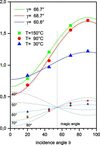Figure 5 The polarization-dependent experimental π* peak intensities (dots) can be mathematically described when for each sample a specific average molecular tilt angle γ is considered. The high-temperature samples show similar behaviour with γ = 66.7° and 68.7°, whereas for T = 30°C the average tilt angle γ = 60.6° is lower and close to the magic angle γmagic = 54.74° with θ independent intensity.

As expected, the mathematical fits (coloured lines) succeed only if, for the three temperatures, the respective fit curves are scaled and different tilt angles are applied (γ = 60.6°, 68.7°, 66.7° ± 0.5°). However, due to the non-molecular fractions mentioned before, the three calculated scaling factors are not equal. Using these factors, the experimental intensities can be scaled (small squares, circles and triangles) to meet the magic angle criterion I(γmagic) = 1/3 (bottom of Fig. 5). Now the three experimental data sets can be compared with the calculated curves (grey lines).

However, the assembly hypothesis mentioned before is not confirmed. In particular, the spectrum of the sample prepared at room temperature, which shows low polarization dependence, suggests that there might be a considerable fraction of disordered molecules. Therefore, we present a possible alternative evaluation procedure, where the peak areas consist of both a constant and a polarization-dependent fraction, which originate from amorphous and crystalline phases, respectively. If for each temperature a certain fraction of randomly oriented molecules is considered, it is possible to allocate a shared tilt angle for all samples (Table 3).

 Table 3In order to allocate a shared tilt angle for the ordered molecular fractions of the three samples, the fitting curves must be scaled and shifted
 The scaling factors correspond to the amount of molecular carbon, whereas the shift constant determines the polarization-independent (disordered) molecular fractions.
 Preparation temperature TS 30° 90° 150° Scaling factor 1.45 ± 0.13 3.90 ± 0.04 3.66 ± 0.19 Shift constant 0.40 ± 0.06 ∼0 0.05 ± 0.02 Polarization-independent fraction 54 ± 15% ∼0 12 ± 5% Tilt angle γ 68.7° 68.7° 68.7°

In that case the fit of the shared tilt angle turned out to be equal to that of the TS = 90°C sample (γ = 68.7°), indicating that at 90°C almost all molecules are well assembled. Indeed, the calculated constant (polarization-independent) intensity fractions of the C1sπ* transitions are 54 ± 15%, 0 ± 4% and 12 ± 5% for TS = 30°, 90° and 150°C, respectively (Fig. 6).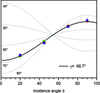Figure 6 The experimental data of Fig. 5have been simulated when non-polarized (constant) fractions of the NEXAFS intensities are considered, which correspond to disordered molecular fractions. The graph shows the polarization-dependent fraction of the three samples, which gives a global tilt angle γ for the three spectra.

### 4. Conclusions

The molecular arrangements of 3 nm ZnPc layers, which had been vacuum thermally deposited on naturally oxidized Si substrates at TS = 30°, 90° and 150°C substrate temperature, have been analysed by NEXAFS spectroscopy at their carbon edges at 287.33 eV. The edge-normalized spectra could be mathematically described when three different carbon fractions were assumed. The analysis distinguishes between well-assembled and disordered ZnPc molecules, as well as non-phthalocyanine carbon. The well-assembled fraction can be mathematically fitted using a global tilt angle of γ = 68.7°. The temperature-dependent disordered fraction, however, averages γ according to its amount, thus giving lower (average) angles for lower temperatures. The third fraction is attributed to organic solvents on the substrates, which can be observed mainly in the TS = 30°C sample. At higher temperatures this fraction is strongly reduced.

### Acknowledgements

We acknowledge Joachim Stöhr for helpful discussions and the ISA-Aarhus for beam time.

### Funding information

The following funding is acknowledged: Bundesministerium für Umwelt, Naturschutz und Reaktorsicherheit (contract No. 03X3525A); Sixth Framework Programme (contract No. RII3-CT-2004-506008).

### ReferencesJOURNAL OFSYNCHROTRONRADIATION